• 典型信号有哪些
千次阅读
2020-09-08 19:04:48

# 典型的数字信号

1.正弦信号

clear all;
close all;
t=(0:0.001:1)';
y=sin(2*pi*30*t)+2*sin(2*pi*60*t);   %原始信号由两个正弦信号叠加产生
randn('state',0);
yn=y+0.5*randn(size(t));      %添加噪声信号
figure;
subplot(121);%图像以1行2列的形式表示，1代表位于第一个位置
plot(t(1:100),y(1:100));
subplot(122);% 图像布局，第二个
plot(t(1:100),yn(1:100));
set(gcf,'position',[300,300,500,500]);% 设置窗口位置2.抽样信号

clear all;
close all;
n=-8:8; % 横坐标设置为从-8~8
y=[zeros(1,13),1,zeros(1,3)];     %产生单位抽样序列
figure;%画图
stem(n,y,'LineWidth',2);
box off;%设置坐标轴边框线
set(gcf,'position',[100,100,500,500]);3.单位阶跃序列

%%  单位阶跃序列
clear all;
close all;
n=-8:8;
yn=[zeros(1,8),ones(1,9)];
figure;
stem(n,yn,'LineWidth',2);
box off;
set(gcf,'position',[100,100,300,200]);4.正弦序列

clear all;
close all;
n=0:40;
x=6*sin(pi*n/4+pi/3);     %幅值是6
figure;
stem(n,x);
set(gcf,'position',[200,200,400,300]);5.实指数序列

%%   实指数序列
clear all;
close all;
n=0:20;
x=(7/3).^n;
figure;
stem(n,x,'filled');
set(gcf,'position',[200,200,500,300]);6.随机序列

%%  随机序列
clear all;
close all;
n=1:30;
x=4+sqrt(2)*randn(1,30);
%randn产生均值为0  方差为1  的随机序列 调整后 均值为4 方差为2
figure;
stem(n,x);
set(gcf,'position',[200,200,400,300]);7.周期序列

%%  周期序列
clear all;
close all;
n1=0:15;
x=(7/4).^n1;        %本来是指数序列
N=10;     %周期
k=5;      %周期的数目
n2=0:(k*N-1);
y=x(mod(n2,N)+1);    %    扩展成5个周期的信号
figure;
stem(n2,y);
set(gcf,'position',[200,200,400,300]);matlab
更多相关内容
• 文章目录一、四类典型信号1.1 连续时间和离散时间的指数信号1.2 连续时间和离散时间的正弦信号1.3 连续时间和离散时间的单位冲激信号和单位阶跃信号1.3.1 单位冲激函数的作用：1.4 Sa信号（sinc信号） 一、四类典型...

# 一、几类典型信号

## 1.1 连续时间和离散时间的指数信号

P a r t 1 Part 1 】：连续时间
首先，表达式就是： x ( t ) = C e a t x(t) = Ce^{at} ，其中，C 和 a 都可以是复数。下面我们先从实指数信号入手：

这一点，我们并不陌生，如果我们把 C 取成 1 ，那么信号就变为： y = e a t y=e^{at} ，对于这个信号，当 a > 0 a>0 时，是单调递增的； a < 0 a<0 时单调递减。

那么接着，我们扩大到复指数信号，如果还是令 C=1，但令 a = j ω 0 a = jω_0 ，那么 x ( t ) = e j ω 0 t x(t) = e^{jω_0t} 。这是一个周期性复指数信号，为什么呢？我们从三个角度来看看：

1. 根据欧拉公式： x ( t ) = e j ω 0 t = c o s ( ω 0 t ) + j s i n ( ω 0 t ) x(t) = e^{jω_0t} = cos(ω_0t) + jsin(ω_0t) ，那么， c o s ( ω 0 t ) cos(ω_0t) s i n ( ω 0 t ) sin(ω_0t) 都是周期信号，周期信号加周期信号依然是周期信号，对吧
2. 我们从严谨的角度证明：假设 x ( t ) x(t) 是周期信号，那么就有： x ( t ) = x ( t + T ) x(t) = x(t+T) ，对于 x ( t + T ) = e j ω 0 ( t + T ) = e j ω 0 t e j ω 0 T = x ( t ) x(t+T) = e^{jω_0(t+T)} = e^{jω_0t}e^{jω_0T} = x(t) ，那应有： e j ω 0 T = 1 e^{jω_0T} = 1 ，根据复变函数的知识， e j 2 k π = 1 e^{j2kπ} = 1 ，故应有： ω 0 T = 2 k π ω_0T = 2kπ ，所以当 T = 2 k π ω 0 T = \frac{2kπ}{ω_0} 时， x ( t ) x(t) 是周期信号，而我们发现 T 可以取任何实数，也就是说 x ( t ) x(t) 就是一个周期信号。
3. 我们可以把 x ( t ) = e j ω 0 t x(t) = e^{jω_0t} 的图像画一下看看效果：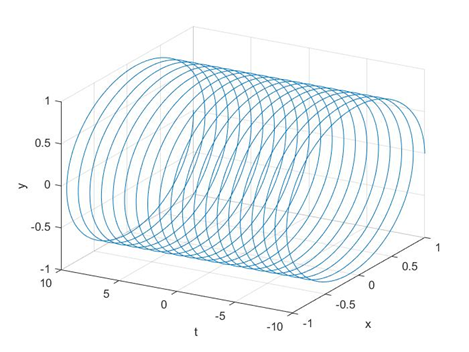P a r t 2 Part 2 】：离散时间
对于离散时间的指数信号，我们这样表示： x [ n ] = C α n x[n] = Cα^n （其中，C，α 可以是复数）

同样地，我们先来分析一下实指数信号。大家注意：离散时间信号的单调性和连续时间是不一样的，当 α > 1时单调递增、0 < α < 1 时单调递减、α<-1时震荡地增大 、-1<α<0 时震荡地减小

我们看看效果图：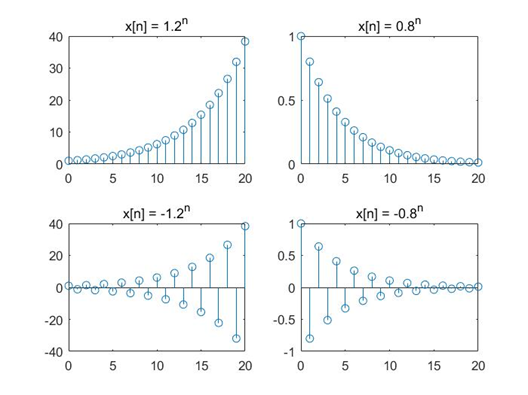好的，现在我们知道实指数下连续时间信号和离散时间信号的单调性的区别了，下面我们看看离散时间下的复指数信号是不是都是周期的呢？？

对于复指数信号，我们也是设： x [ n ] = e j ω 0 n x[n] = e^{jω_0n}
类似地，我们假设存在这样一个周期 N，有： x [ n ] = x [ n + N ] x[n] = x[n+N] ，即： x [ n + N ] = e j ω 0 ( n + N ) = e j ω 0 n e j ω 0 N x[n+N] = e^{jω_0(n+N)} = e^{jω_0n}e^{jω_0N} ，所以应有： e j ω 0 N = 1 e^{jω_0N} = 1 ，根据之前的推论，我们有： N = 2 m π ω 0 N = \frac{2mπ}{ω_0} 现在问题来了：我们知道离散时间信号的横坐标都是整数！所以周期 N 应该也是整数才对，可是这里有一个 π π ，所以这就要求 ω 0 ω_0 应该有个 π π ，这样N才有可能是整数

所以离散时间的复指数信号不一定是周期函数！下图说明：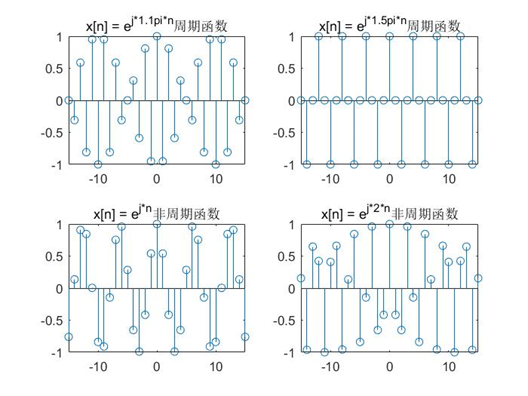另外，在计算离散时间复指数信号的基波周期时，需要先把 m m 带上，因为 2 π 2π 除以 ω 0 ω_0 得到的不一定是整数（虽然 π π 抵消掉了），可能是分数，所以需要有一个 m 将N 变成整数

## 1.2 连续时间和离散时间的正弦信号

这里关于连续时间的正弦信号就不写了，基本没啥问题。主要是在于离散时间信号下的正弦信号。

注意： 离散时间的正弦信号不一定是周期的！！
比如 x [ n ] = c o s ( ω 0 n ) x[n] = cos(ω_0n) ，只有当 2 Π ω 0 \frac{2Π}{ω_0} 是整数时，这个信号才是周期的

## 1.3 连续时间和离散时间的单位冲激信号和单位阶跃信号

P a r t 1 Part 1 】：离散时间
我们先看看离散时间下单位冲激函数的定义： δ [ n ] = { 1 , n = 0 0 , n ≠ 0 δ[n]= \begin{cases} 1, n=0\\ 0, n≠0 \end{cases}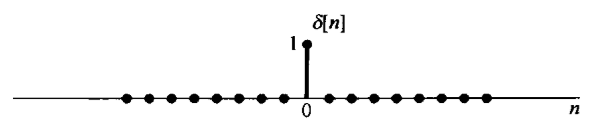再来看看单位阶跃函数的定义： u [ n ] = { 1 , n ≥ 0 0 , n < 0 u[n]= \begin{cases} 1,n≥0\\ 0,n<0\\ \end{cases}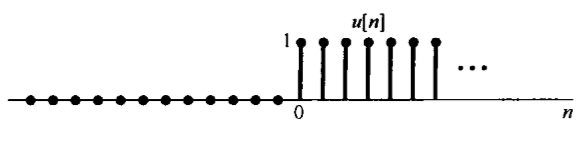下面，我们重点分析一下这两个函数的关系：
首先，从 u [ n ] u[n] 的角度， δ [ n ] δ[n] 可以看成是 u [ n ] u[n] 的一阶差分： δ [ n ] = u [ n ] − u [ n − 1 ] δ[n] = u[n] - u[n-1]

而反过来，从 δ [ n ] δ[n] 的角度，我们也可以得到： u [ n ] = ∑ k = 0 ∞ δ [ n − k ] = ∑ k = − ∞ n δ [ k ] u[n] = \sum_{k=0}^{∞}δ[n-k] = \sum_{k=-∞}^{n}δ[k]

我们下面来解释一下：对于上式的第一个等号，应该很好理解，就是一个 δ [ n ] δ[n] 函数不断右移累加的过程，那么第二个等号呢？

这时，我们要记住一点：此时的横轴依然是 n 轴，而 k 只是 n 轴上的一点，这时假设 n < 0，那么自变量从 k 一直取到 n 都是 0，那么累加的结果也就是0（得到：n < 0时，结果=0）；如果 n> 0，那么结果中始终有1，得到（n > 0时，结果=1）。这正好满足单位阶跃函数的定义。

如下图所示：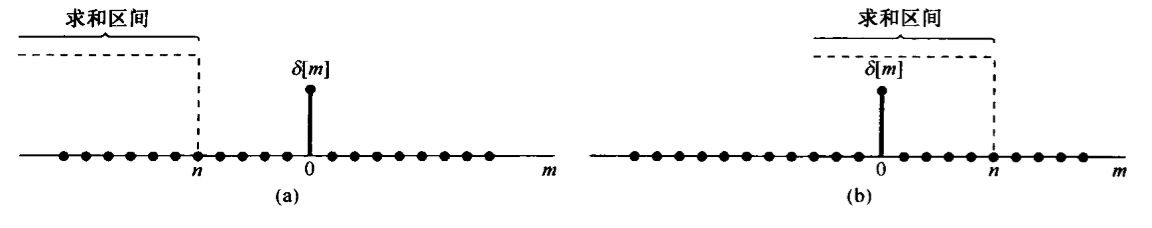P a r t 2 Part 2 】连续时间
这里，我们先定义一下连续时间的单位阶跃函数： u ( t ) = { 0 , t < 0 1 , t > 0 u(t) = \begin{cases} 0, t <0\\ 1,t>0\\ \end{cases}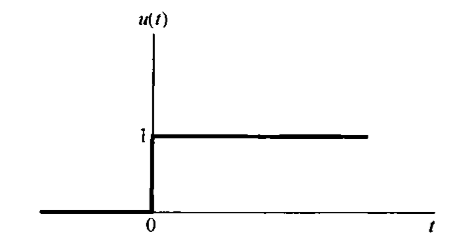这个时候，我们想起来一件事情：在离散时间信号下，我们可以通过对阶跃函数做一阶差分，得到单位冲激函数。那么，应该类似地，在连续时间信号下，如果对阶跃函数求一阶微分，那么是不是就能够得到单位冲激函数了呢？

可是现在遇到了一个问题，就是 u ( t ) u(t) 在 t = 0处是间断的，不连续，不可导。那怎么办呢？我们可以给 u ( t ) u(t) 在 t = 0处一个小的时间间隔 △ △ ，让函数在这个小的时间间隔里面 从0变到1，如下图所示：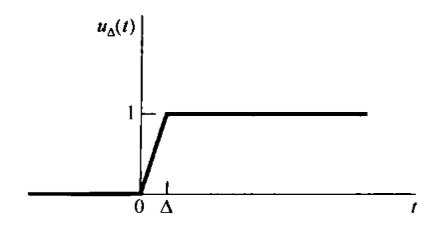你看，这样不就可导了么！这个函数 u △ ( t ) u_{△}(t) 的导数就是 在 0 0 ~ △ △ 处是 1 △ \frac{1}{△} ，其他地方是0，也就是说：导数的结果是一个宽度为： △ △ ，高度是 1 △ \frac{1}{△} 的矩形：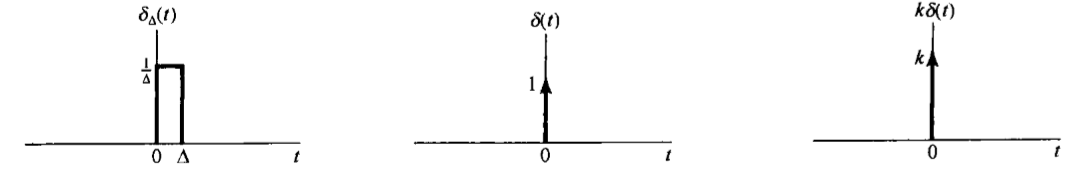如果 △ △ 不断减小，一直逼近0，那么，最终这个函数就会变成一条直线，那么我们要注意：连续时间下的单位冲激函数是一个面积函数！

而且我们还可以知道，这个单位冲激函数在整个事件轴上的积分——也就是它的面积，始终是 1 ！
∫ − ∞ + ∞ δ ( t ) d t = 1 \int_{-∞}^{+∞}δ(t)dt = 1
当然啦，无论你怎么平移这个函数，它在整个时间轴上的积分都是1： ∫ − ∞ + ∞ δ ( t − t 0 ) d t = 1 \int_{-∞}^{+∞}δ(t-t_0)dt = 1

经过上面的分析：我们可以得到一个不太严谨但是很重要的思想：对于一个间断函数求微分，在中断点处将会得到一个冲激函数：冲激函数的幅度就是原函数跳变的幅度、冲激函数箭头的方向就是原函数的跳变方向（从大跳变到小：箭头向下、从小跳变到大：箭头向上）

画图的时候大家千万要注意：如果原信号有跳变的，那么对他微分之后，那个跳变的地方是需要画一个冲激的！！！

同样地、反过来通过 δ ( t ) δ(t) 怎么推出 u ( t ) u(t) 呢？ u ( t ) = ∫ 0 + ∞ δ ( t − τ ) d τ = ∫ − ∞ t δ ( т ) d τ u(t) = \int_{0}^{+∞}δ(t-τ)dτ = \int_{-∞}^tδ(т)dτ

对于第二个等号，我们也是通过积分区间来分析的：假如 t < 0 t <0 ：那么你从负无穷开始积到 t ，无论你怎么积，都是0，但是如果 t > 0 t > 0 ，那么你的积分始终是1，这满足阶跃函数的定义。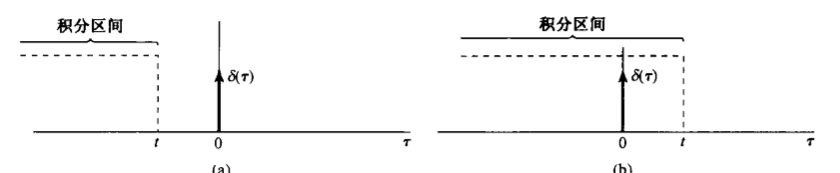### 1.3.1 单位冲激函数的作用：

既然我们引入了单位冲激函数和单位阶跃函数，并把他们作为典型信号，那么它们肯定是有一些有用的作用的，我们来看看：

单位冲击函数，无论是离散时间的还是连续时间的，都具有抽样的功能！

也就是说，对于某一个信号 x ( t ) x(t) ，如果用 δ ( t ) δ(t) 来抽样，就是： x ( t ) δ ( t ) = x ( 0 ) δ ( t ) x(t)δ(t) = x(0)δ(t)
也即是抽取了 x ( t ) x(t) 函数 t = 0 t=0 处的信号。如果想要抽取其他某一时刻的信号，就是： x ( t ) δ ( t − t 0 ) = x ( t 0 ) δ ( t − t 0 ) x(t)δ(t-t_0) = x(t_0)δ(t-t_0)
注意： x ( t ) δ ( t ) ≠ x ( 0 ) x(t)δ(t) ≠ x(0) ！！！因为 x ( t ) δ ( t ) x(t)δ(t) 是一个信号图形，而 x ( 0 ) x(0) 是一个值。

下面课堂上提出了一个有趣的例题：

1. 计算积分： ∫ − 5 5 c o s ( 2 Π t ) δ ( t + 3 ) d t \int_{-5}^5cos(2Πt)δ(t+3)dt
首先，我们看积分项： c o s ( 2 Π t ) δ ( t + 3 ) cos(2Πt)δ(t+3) ，这个信号 c o s ( 2 Π t ) cos(2Πt) δ ( t + 3 ) δ(t+3) 相乘，就相当于采样了 c o s ( 2 Π t ) cos(2Πt) 在 t = -3 时的值，即 c o s ( 2 Π t ) δ ( t + 3 ) = c o s ( − 2 Π 3 ) δ ( t + 3 ) = c o s ( 6 Π ) δ ( t + 3 ) = δ ( t + 3 ) cos(2Πt)δ(t+3) = cos(-2Π3)δ(t+3) = cos(6Π)δ(t+3) = δ(t+3)
下面，我们就看看积分区间，[-5 , 5] 包括了 t = -3 的地方，因此，积分区间下函数的面积就是1 ，因此答案就是 1

## 1.4 矩形信号（门信号）

矩形信号如下图所示，它只有在某一段时间内有幅值，其他时间都是0，如下图：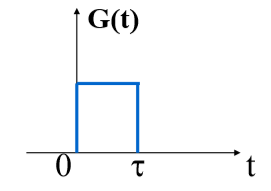这个矩形信号，其实我们可以用阶跃函数表示出来。

比如上图的矩形信号，我们就可以表示成： G ( t ) = u ( t ) − u ( t − τ ) G(t) = u(t) - u(t-τ)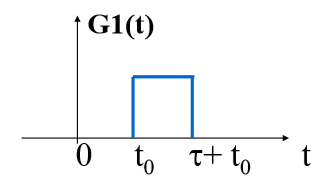这就可以表示成： u ( t − t 0 ) − u ( t − t 0 − τ ) u(t-t_0) - u(t-t_0-τ)

## 1.4 Sa信号（sinc信号）

Sa信号在我的这一篇 B l o g Blog 中有介绍，这里不再赘述：
【信号与系统学习笔记】—— 傅里叶变换1 从零开始推导傅里叶变换（详细易懂版）

展开全文• 一阶系统的时域分析前言典型输入信号选取测试信号时必须考虑的原则:1.单位脉冲信号2.单位阶跃信号3.单位斜坡信号（速度阶跃信号）4.单位抛物线信号（加速度阶跃信号）5.正弦信号典型时间响应动态过程稳态过程控制...

# 前言

前面学习了控制系统的数学模型。分析、研究和设计控制系统的首要工作是确定系统的数学模型，一旦获得系统的数学模型，便可以用不同的方法分析控制系统的性能。

时域分析法是根据描述系统的微分方程或传递函数，直接求解出在某种典型输入作用下，系统输出量随时间t变化的表达式，即时间相应。然后根据此表达式或其相应的描述曲线来分析系统的稳定性、快速性和准确性。

时域分析法具有直观、准确的优点，并且可以提供系统时间响应的全部信息。

# 典型输入信号

为了求解系统的时间相应，必须已知输入信号的解析表达式。

在分析和研究控制系统时，需要选取一些具有代表性的实验信号作为系统的输入，称为典型输入信号。

## 选取测试信号时必须考虑的原则:

• 选取的输入信号的典型形式应反映系统工作时的大部分实际情况。
• 选取外加输入信号的形式应尽可能简单，易于在实验室获得，以便于数学分析和实验研究。
• 应选取那些能使系统工作在最不利情况下的输入信号作为典型的测试信号。

## 1.单位脉冲信号幅值为无穷大、持续时间为零的理想脉冲信号实际上是无法得到的。脉冲宽度很窄的电压信号、瞬间作用的冲击力等都可以近似为脉冲信号。

单位脉冲信号可以用于考察系统在脉冲扰动后的恢复运动。

## 2.单位阶跃信号单位阶跃信号表示在t=0处，输入量的幅值瞬间由0突变为1的过程。

若信号突变的幅值不为1，如 r ( t ) = R 1 ( t ) r(t) = R 1(t) ，则表示信号为幅值等于R的阶跃函数，其中R为常量。

时域分析中，单位阶跃信号用得最为广泛，实际电路中，开关的转换、电源的突然接通、负荷的突变等，均可视为阶跃信号。

单位阶跃信号可用做考察系统对恒值信号的跟踪能力时的输入信号。

## 3.单位斜坡信号（速度阶跃信号）单位斜坡信号表示由零值开始随时间t线性增长的信号，其斜率为1.

若斜率不为1，如 r ( t ) = R t r(t) = R t ，则表示信号为斜率等于R的斜坡信号。

在闸门均速升降、工件匀速运动时，其位置信号均可视为斜坡信号。

单位斜坡信号是用于考查系统对匀速信号跟踪能力时的实验信号。

## 4.单位抛物线信号（加速度阶跃信号）单位抛物线信号表示信号的大小随时间t以加速度为1的等加速度增加。

以在、恒转矩启动的电动机位置信号，可视为等加速度信号。

单位抛物线信号是用于考察系统对于等加速度信号的跟踪能力时的实验信号。

## 5.正弦信号# 典型时间响应初始状态为零的控制系统，在典型输入信号作用下的输出，称为典型时间响应。

通常，把控制系统的时间响应按时间的顺序划分为动态过程和稳态过程两部分。

## 动态过程

动态过程也称为暂态过程或过渡过程，指系统在典型输入信号作用下，系统输出量从初始状态到最终状态的过程。根据系统结构和参数选择的情况，动态过程表现为衰减、发散和等幅振荡几种形态。

显然，一个可以正常运行的控制系统，其动态过程必须是衰减的，即系统必须是稳定的。

## 稳态过程

稳态过程也称为系统的稳态响应，指系统在典型输入信号作用下，当时间t趋于无穷大时，系统输出量的表现形式。

稳态过程表征系统输出量最终复现输入量的程度，提供系统稳态误差的信息，用系统的稳态性能描述。

实际应用中认为，当系统的输出对其输入的复现进入允许的误差范围后，系统即进入稳态。

# 控制系统的性能指标

## 动态性能指标

描述稳定的系统在单位阶跃信号作用下，动态过程随时间t变化的指标，称为动态性能指标。

通常以阶跃响应来衡量系统控制性能的优劣和定义瞬态过程的时域性能指标。稳定的系统(不计扰动)的单位阶跃响应函数有衰减振荡单调变化两种。

### 衰减振荡#### 1.延迟时间 t d t_d

输出响应第一次达到稳态值的50%所需的时间。

#### 2.上升时间 t r t_r

输出响应第一次达到稳态值y(∞)所需的时间。或指由稳态值的10%上升到稳态值的90%所需的时间。

#### 3.峰值时间 t p t_p

输出响应超过稳态值达到第一个峰值 y m a x y_{max} 所需要的时间。

#### 4.最大超调量(简称超调量) δ δ

瞬态过程中输出响应的最大值超过稳态值的百分数。#### 5.调节时间 t s t_s

当y(t)和y(∞)之间的误差达到规定的范围之内[一般取y(∞)的士5%或土2%,称允许误差范围，用D表示]且以后不再超出此范围的最小时间。即当t≥t,有:#### 6.振荡次数 N N### 单调变化这种系统就无需采用峰值时间和最大超调量这两个指标。

此时最常用的是调节时间这一指标来表示瞬态过程的快速性。

有时也采用上升时间这一指标。

## 稳态性能指标

事实上，当响应时间 t > t s t > t_s 时，系统的输出响应就进入了稳态过程。

稳态误差是描述系统稳态性能的一种性能指标，它是指t趋于无穷时，系统期望输出与实际输出之间的差值

稳态误差 e s s e_{ss} 越小，系统的控制精度就越高。

展开全文信号 时域 性能指标
• 编制确定信号和随机信号的 M 自定义函数文件，MySin.m产生正弦信号。 矩 形 脉 冲 信 号 (MyImpulse)，指数衰减正弦信号(MyExpSin)。
• 三角波信号(MyTri)，方波信号 (MySquare)； 准 周 期 信 号 (MyStdPeriod) ，编制确定信号和的 M 自定义函数文件。
• 各种不同的信号产生方式，以及相关MATLAB程序
• 信号与系统考点归纳与典型题（含考研真题）详解.pdf
• 本文介绍了信号完整性设计中的5个典型问题，对于信号完整性设计学习一定指导意义。
• 关于典型雷达信号的侦察分析和雷达干扰建模与仿真，为雷达系统仿真提供了重要的模块。
• 这一部分我们介绍三个点，如图所示下面分别介绍连续时不变特征信号线性时不变(linear time-invariant)系统特征函数这一系统的信号在微分和积分操作下，函数形式保持不变。对于这样的函数我们称之为该系统的特征函数...

这一部分我们介绍三个点，如图所示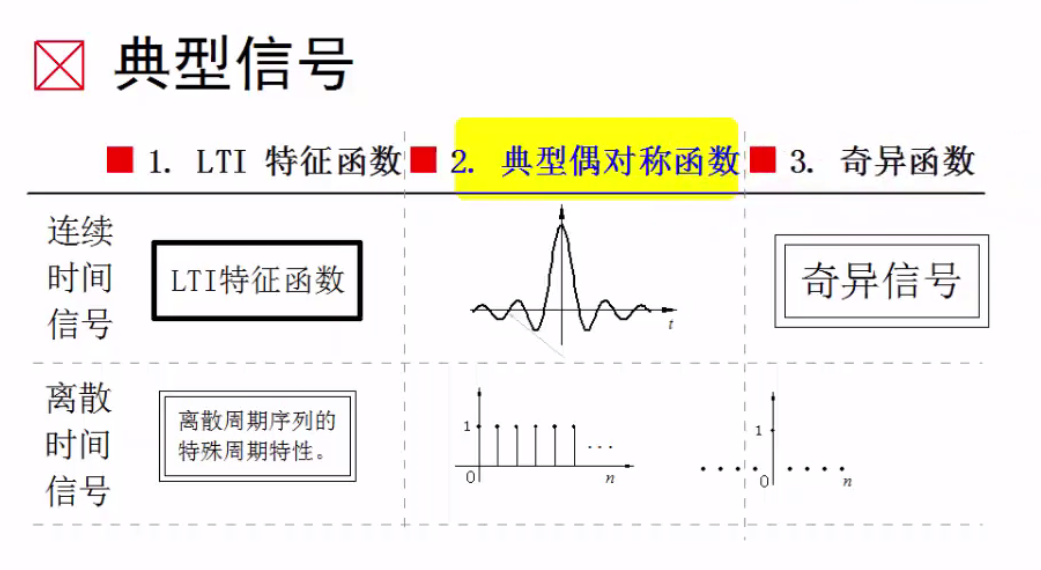下面分别介绍

## 连续时不变特征信号

### 线性时不变(linear time-invariant)系统特征函数

这一系统的信号在微分和积分操作下，函数形式保持不变。对于这样的函数我们称之为该系统的特征函数。我们在理解时可以对比矩阵的特征值和特征向量

Ax=λx

A是某种操作，在这种操作下，特征值和特征向量保持不变。我们还可以想想指数函数，指数函数在微分下指数形式是保持不变的。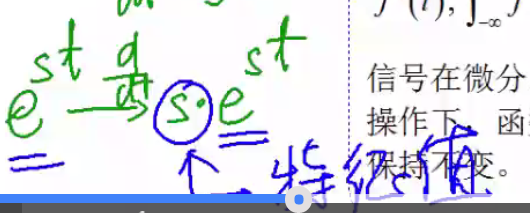一个图概括如下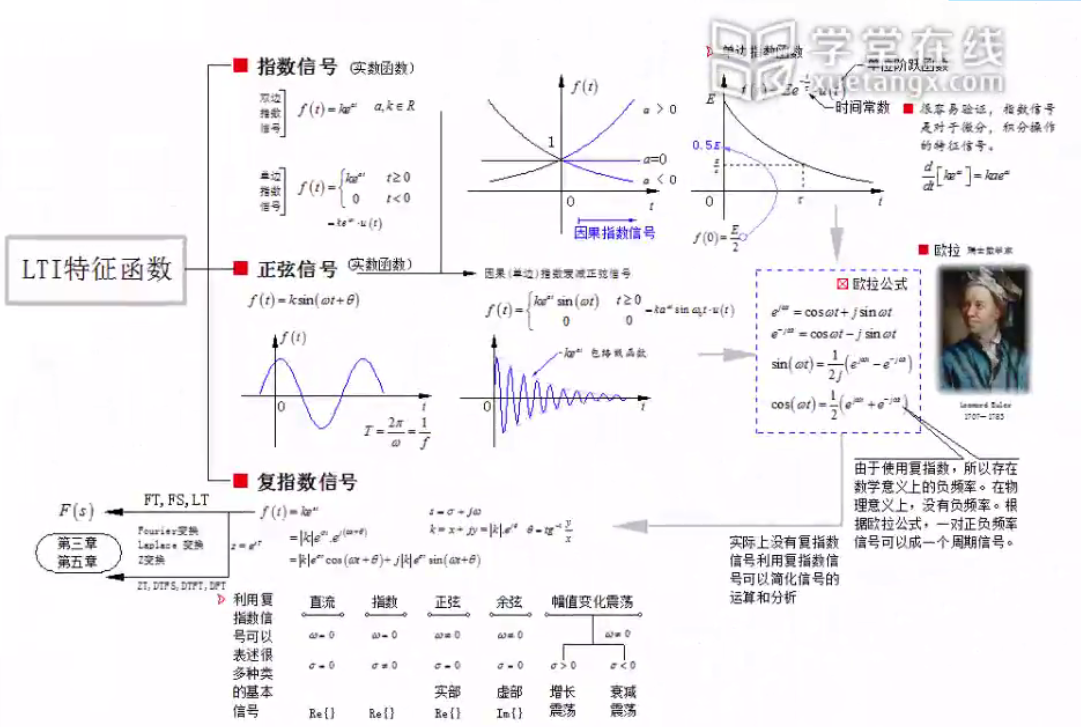## 对称信号

我们在这里介绍的对称信号实际上是一对，他们分别是高斯信号和抽样信号

### 高斯信号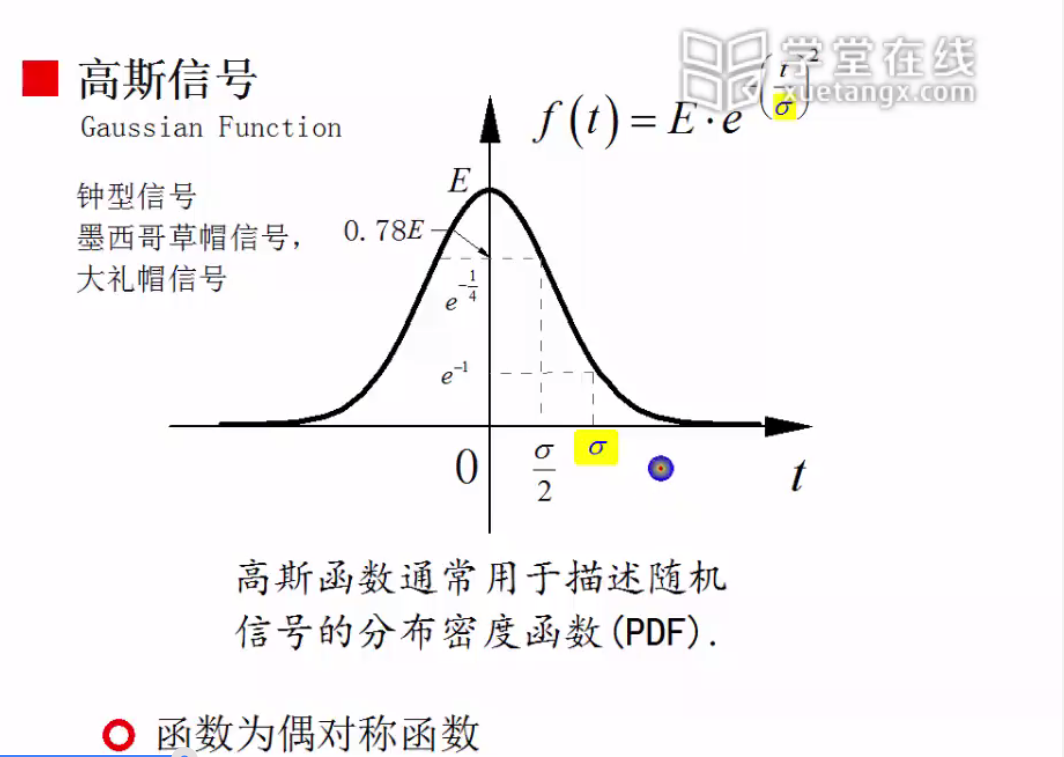注意，高斯信号实际上是一个从负无穷到正无穷一直都存在的信号。但我们知道指数衰减是一种很快的衰减，因此我们可以认为整个信号有效值基本集中在3 σ $\sigma$之间。

函数的性质如图所示：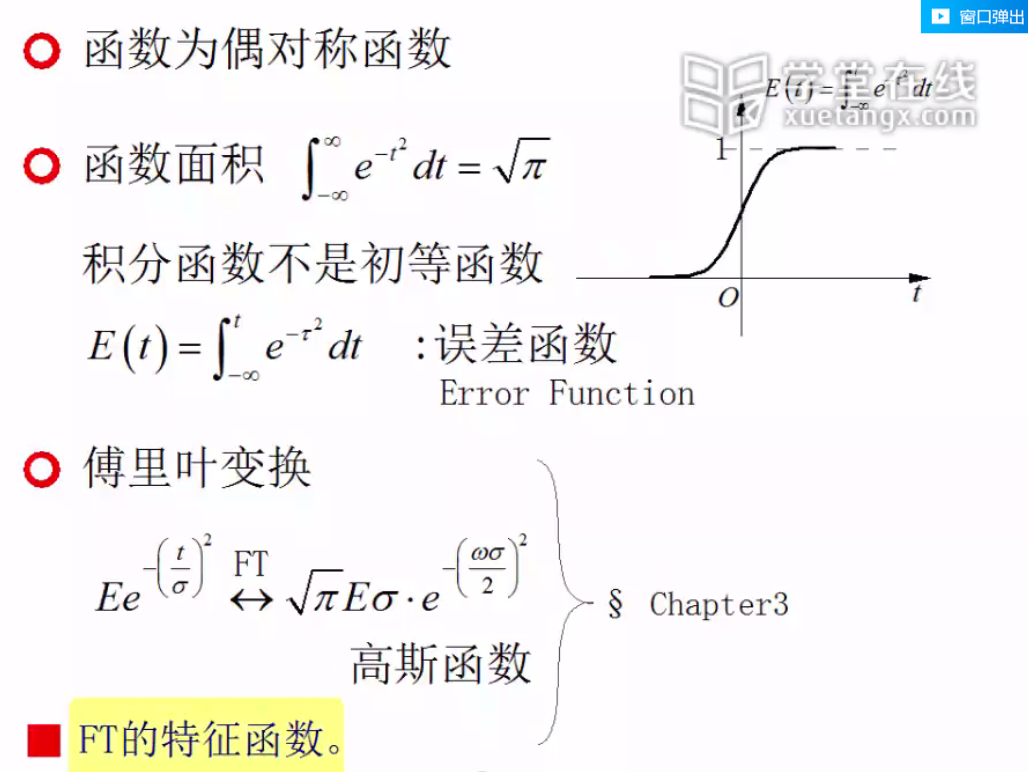### 抽样信号

如图所示为抽样信号的函数形式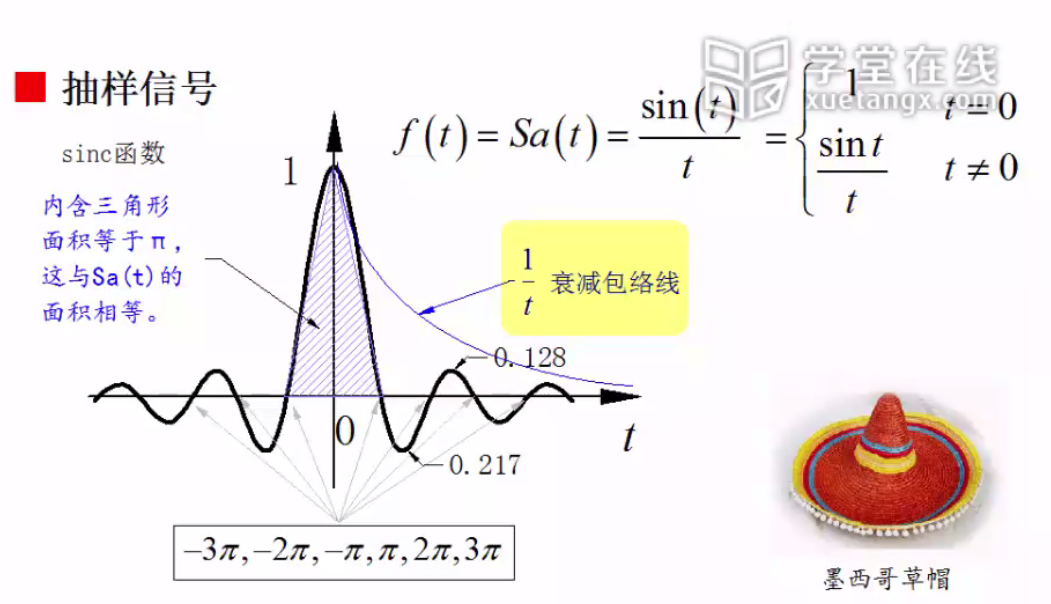函数的性质如下图所示：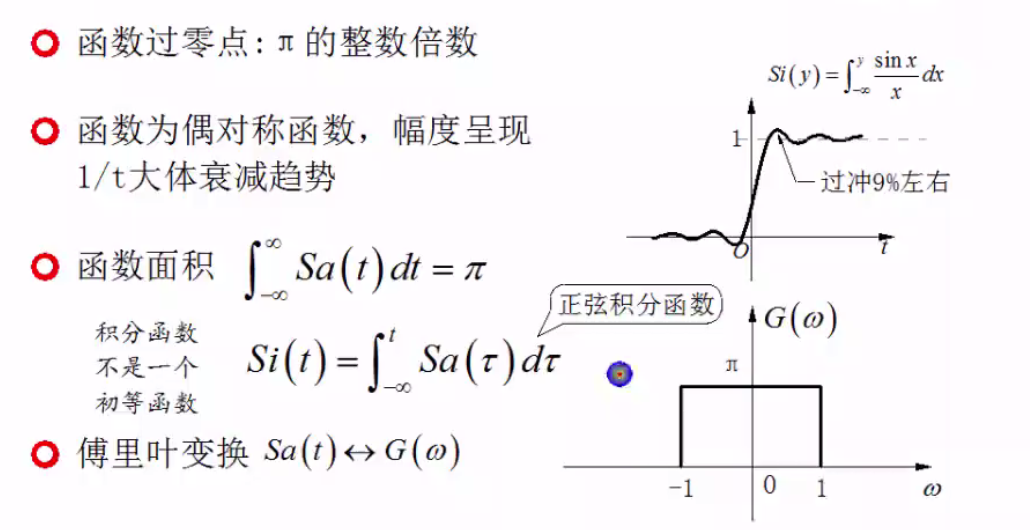# 奇异信号

## 奇异信号介绍

奇异信号是指函数本身或者导数存在不连续点的信号

### 单位斜变信号

如图所示，之所以称斜变信号为奇异信号是因为他的导数是不连续的，负半轴为0正半轴为1.斜变信号有很多应用，最常见的就是老式电视的锯齿形扫描函数。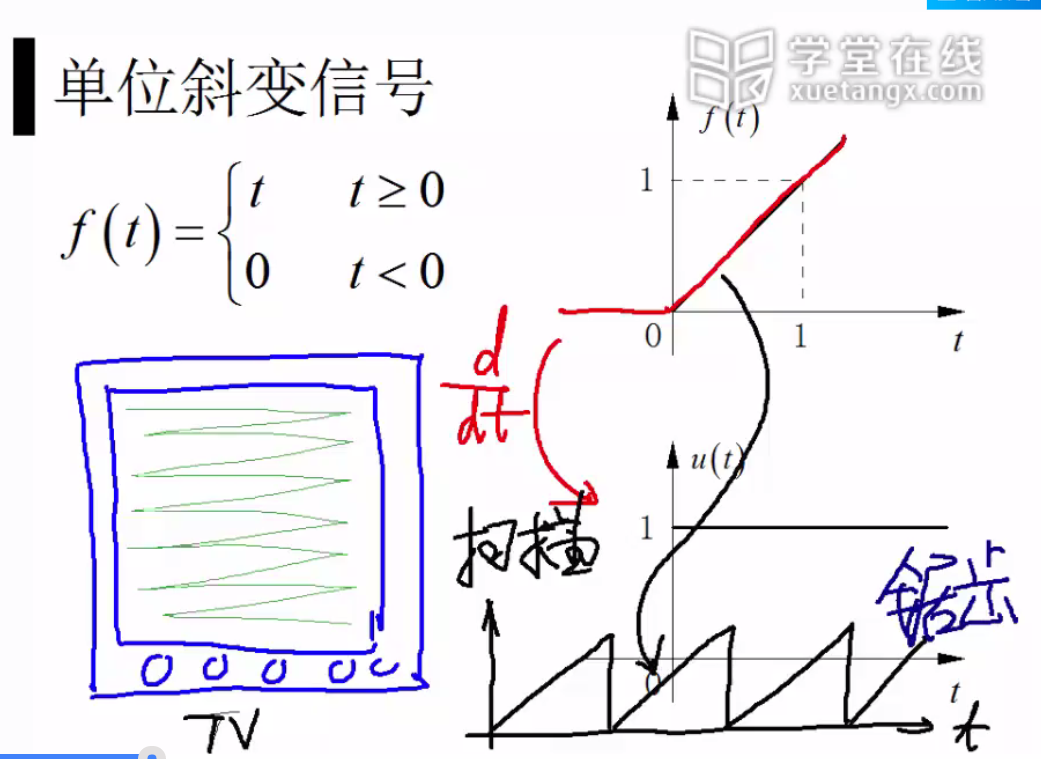### 多项式因果信号

与斜变信号对应的是多项式因果信号，这些多项式信号经过多次求导之后也会变为不连续的信号。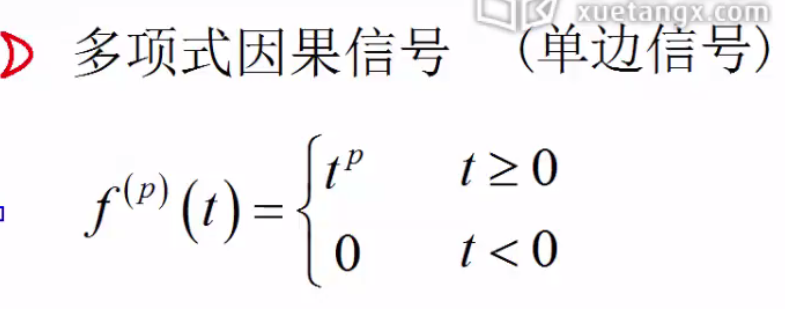### 单位阶跃信号

单位阶跃信号是单位斜变信号求导而得出的结果.我们通常认为在0点处对应的幅值为0.5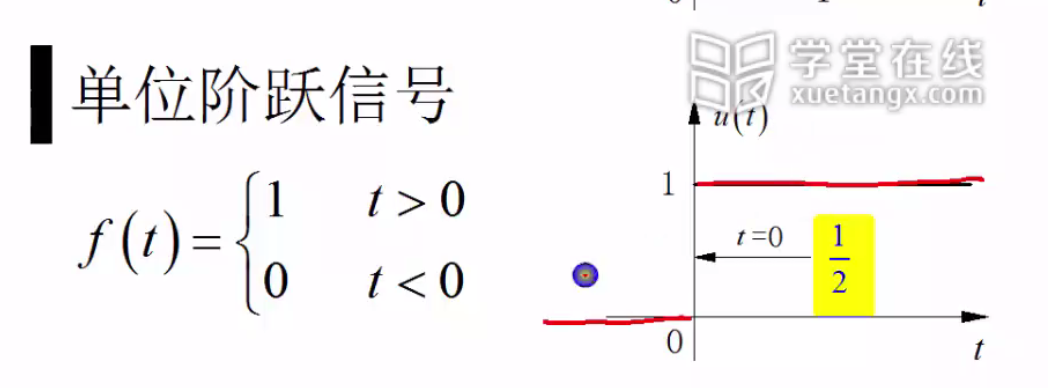### 单位冲击信号

单位阶跃信号再求导就得到了单位冲击信号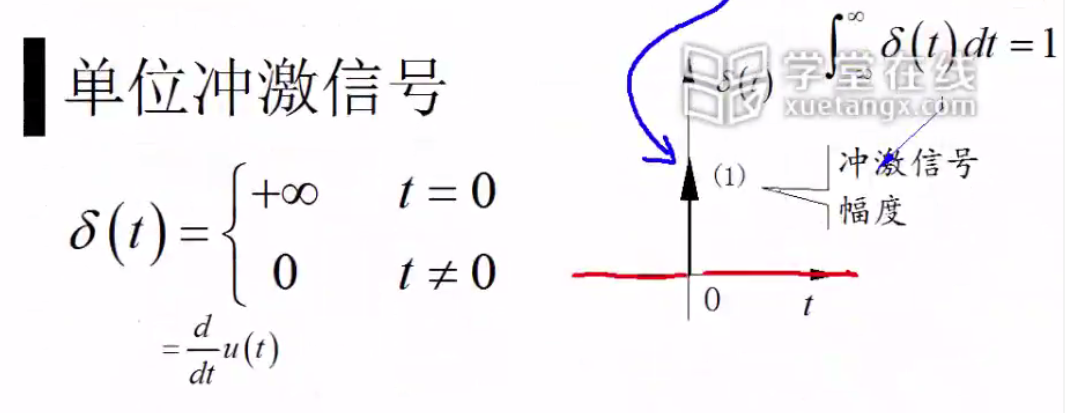### 单位冲击偶信号

我们在单位冲击信号的基础之上再求导，就得到了单位冲击偶信号。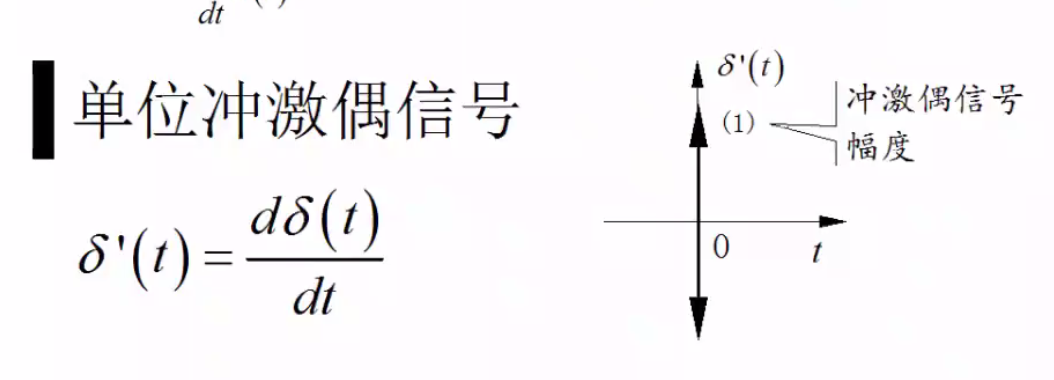最后我们用一张图来概括一下这四类信号，他们之间的关系是逐一求导。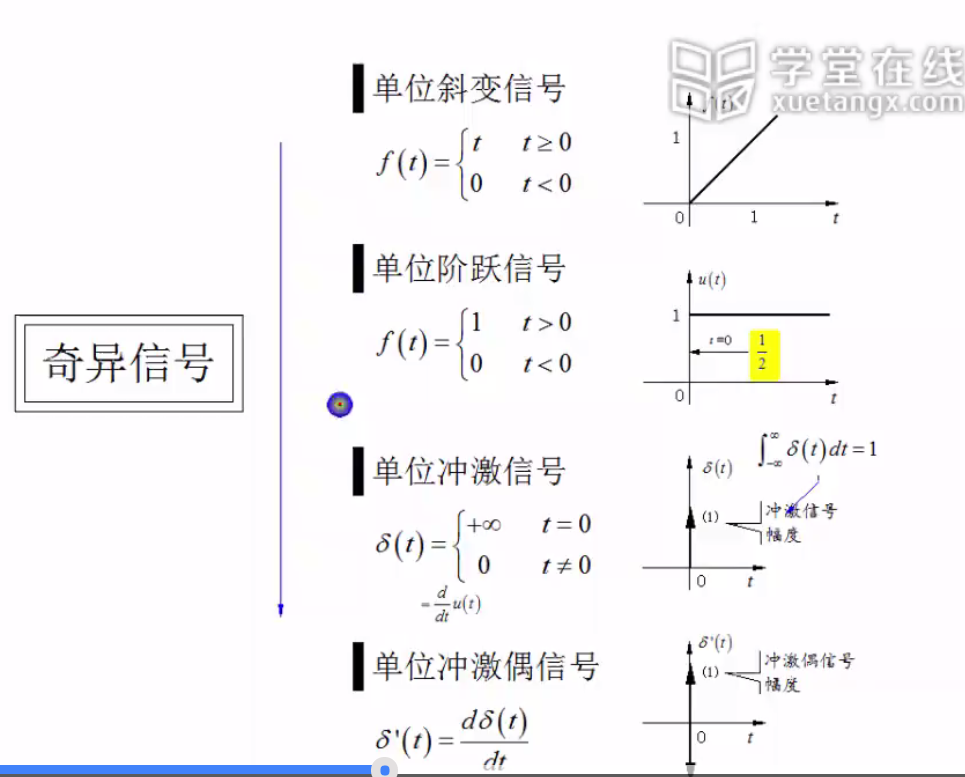### 单位阶跃信号的作用

他与其他信号相乘可以表示信号的起始和结束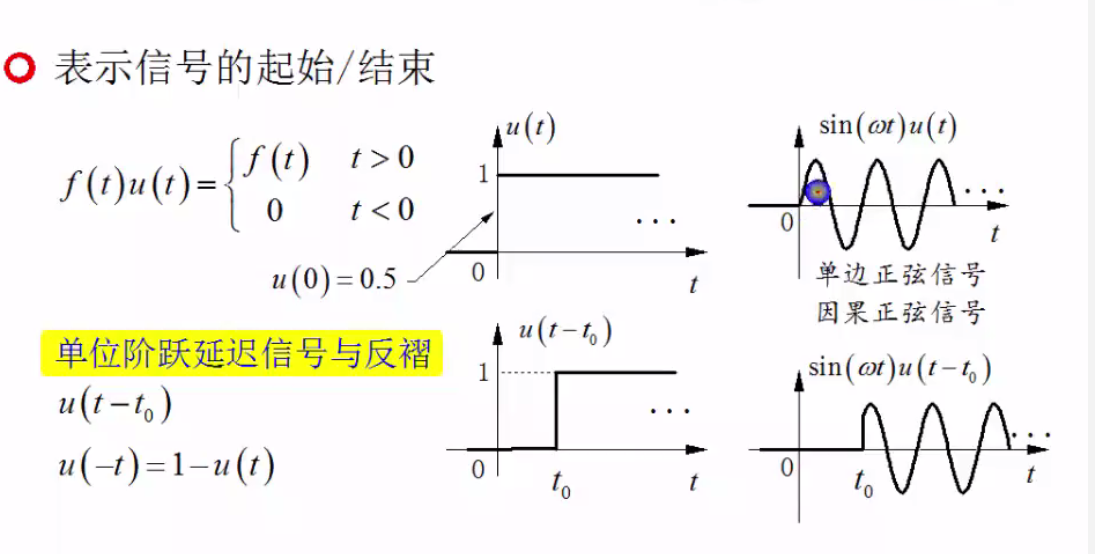也可以和自身相加减之后形成窗口信号，可以用窗口信号来截取别的信号。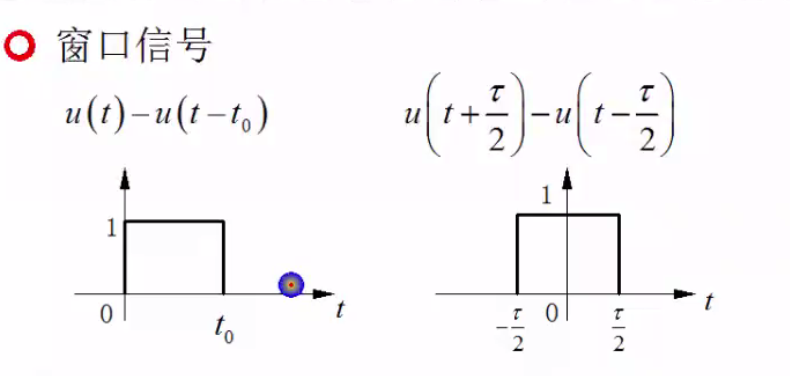下图为截取信号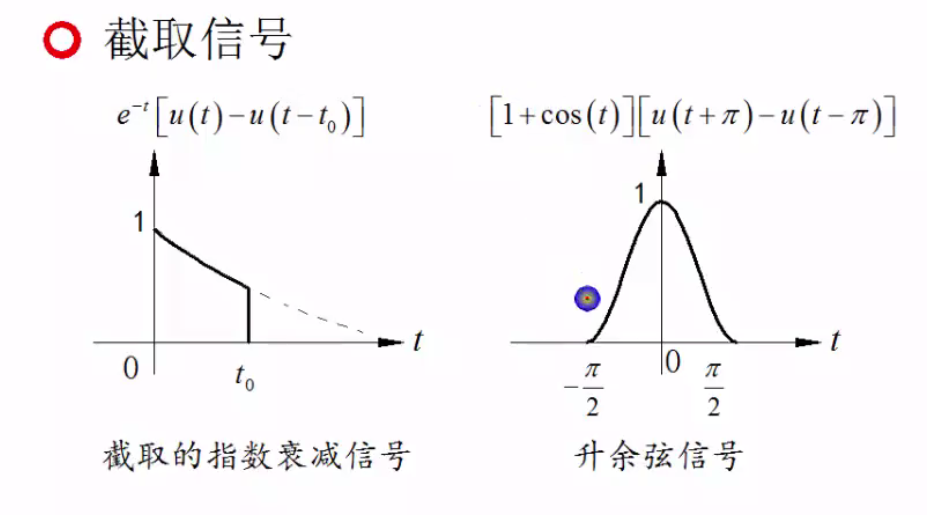在窗口信号的基础之上，我们可以进一步的形成分段信号，分段信号可以写成窗口信号的组合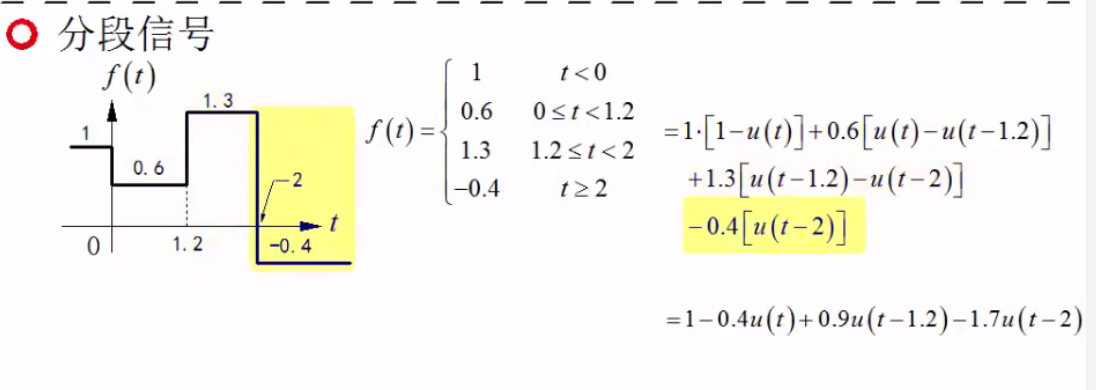单位阶跃信号还和符号函数有着密切的关系，如图所示，二者实际上是满足线性关系的。

## 连续时间奇异信号

虽然单位冲激函数是单位阶跃函数的导数，但是不是很好理解，我们可以这样理解。想象一个面积为1的长方形，当他的底为0的时候高度为无穷大。

因为单位冲激函数和狄克拉函数的定义一样，所以我们有的时候也称单位冲激函数为derda函数。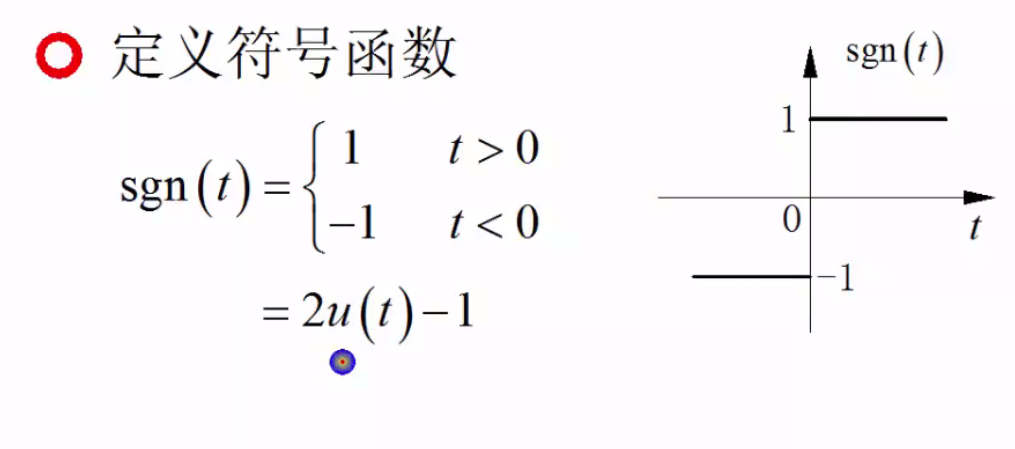### 单位冲击信号的数学特性

如图所示，单位冲击信号与任意信号的积分实际上是任意信号在零点的取值。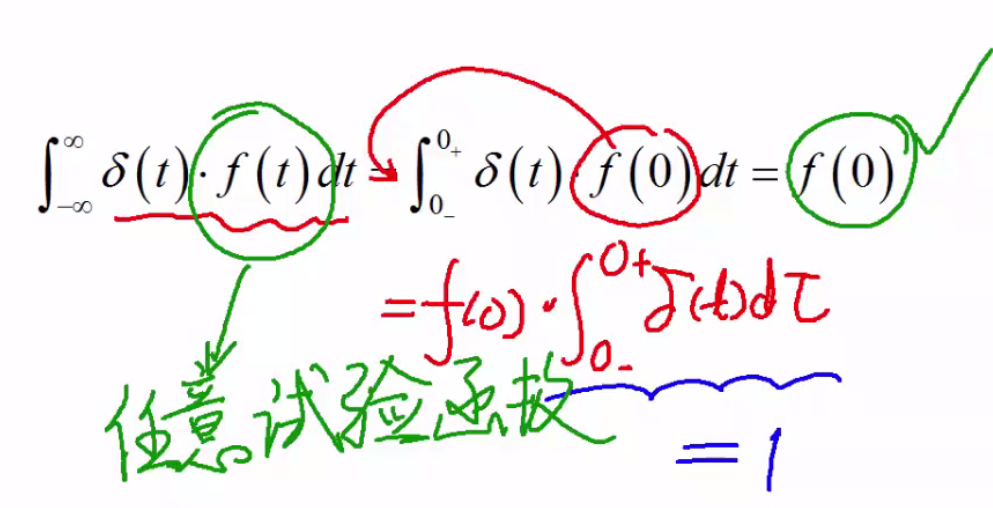我们根据此公式反过来也可以证明冲击信号，也就是满足这个条件的信号就是冲击信号。

他还有这样的性质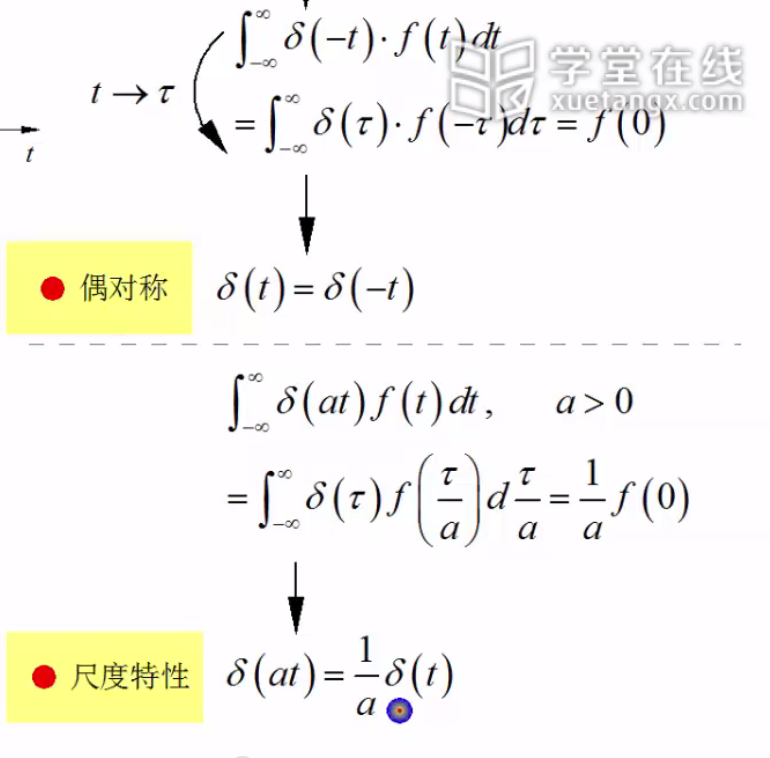如下图所示，可以进行这样的变换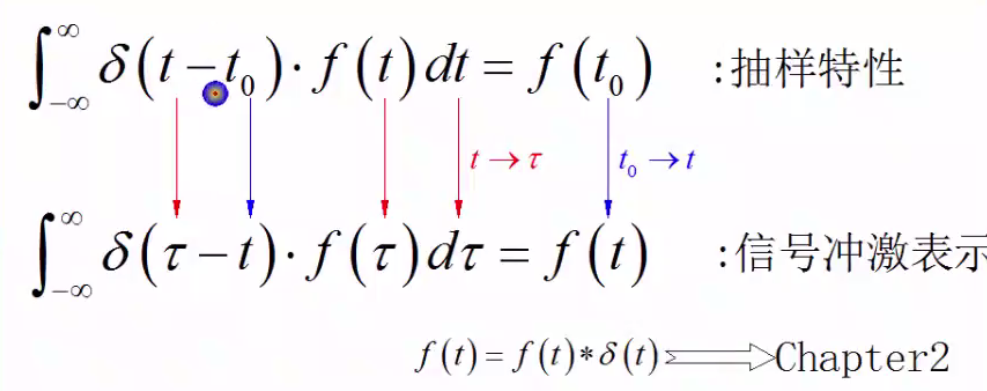最后我们总结一下冲激函数和冲击偶函数的性质：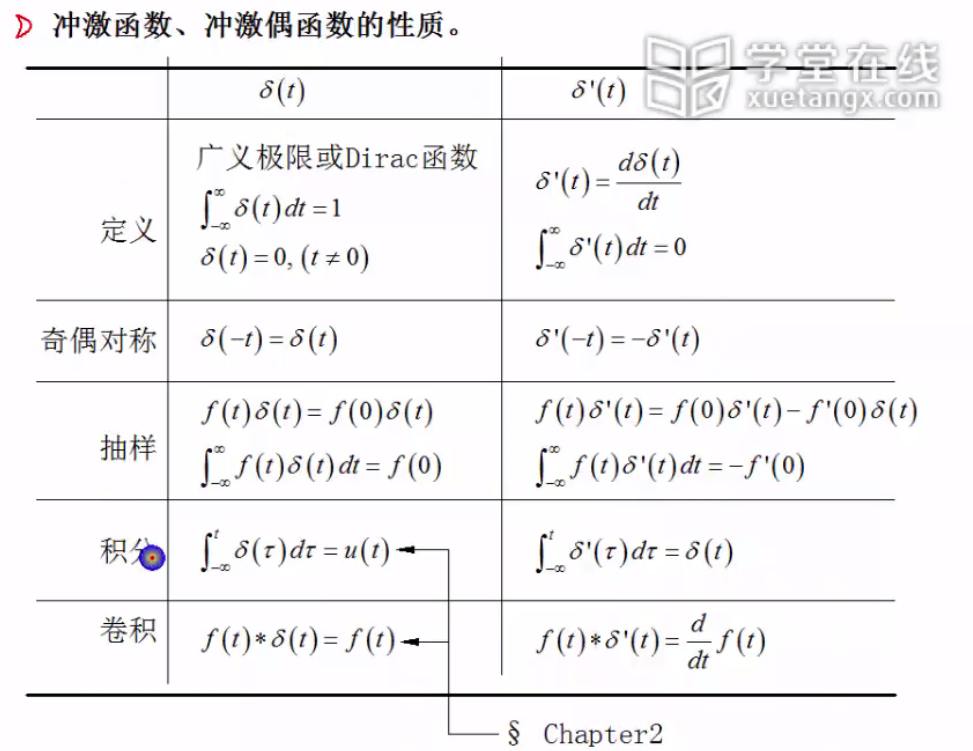## 离散时间奇异信号

对于离散时间信号我们和连续时间信号比较着来学习如图所示，二者之间可以相互转化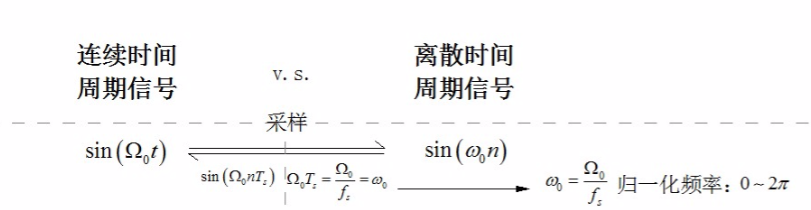如下图所示，对于连续周期信号而言，他总是周期信号。但是对于序列信号不一定。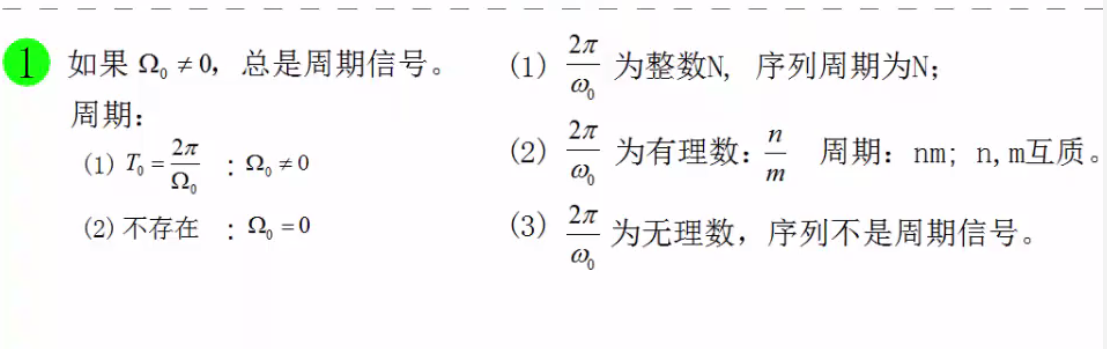序列信号可以额看成是周期的包络线信号和周期的脉冲信号的乘积。两个周期信号的复合信号有可能是一个周期信号，也有可能是一个非周期的信号。对于序列信号来说，只有当他的频率与 π $\pi$的比值为有理数的时候，他才是严格意义上的周期信号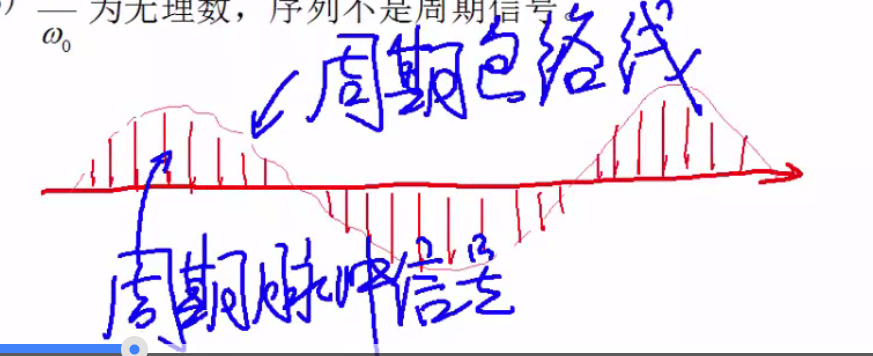对于连续周期信号我们知道随着频率的增加，他的波形变得越来越密。但是对于离散周期信号并不是这样。如图所示，随著频率的增加，它展现了一种周期性。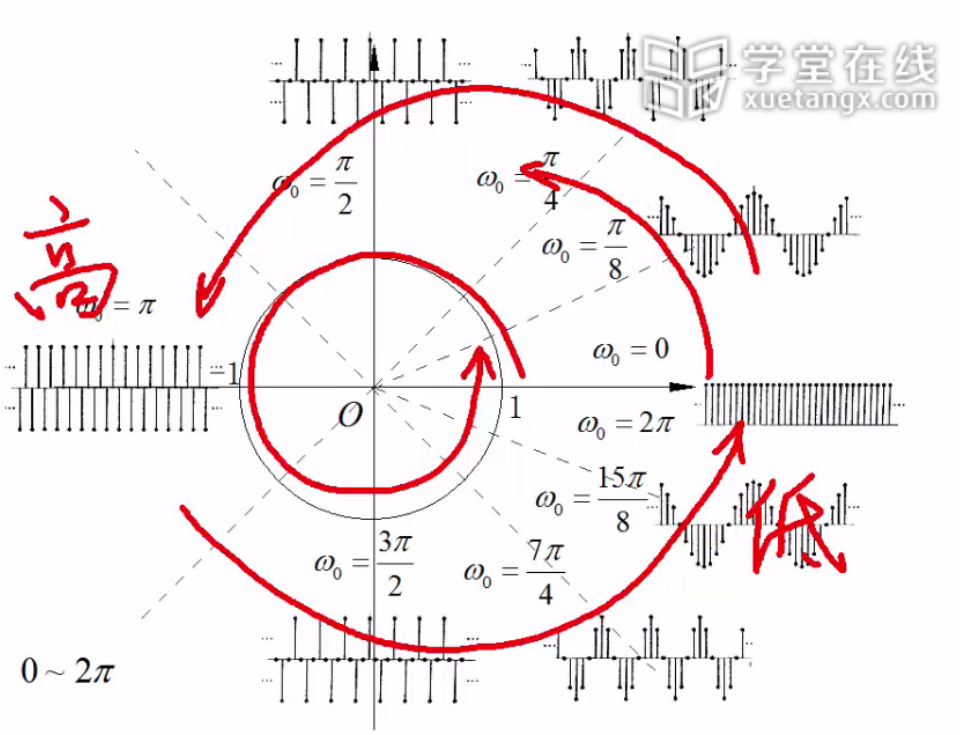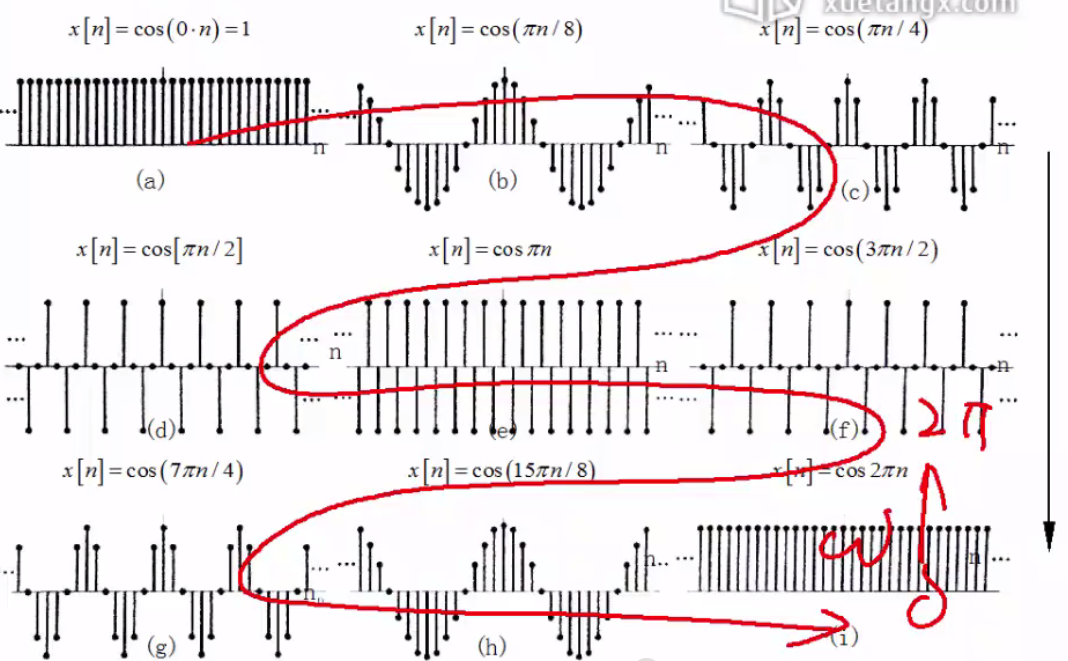另外对于连续的单位冲击信号，在0点的取值是无穷大，但是对于离散的信号序列而言是1.连续的单位阶跃信号在0点的取值是1/2，离散的信号序列在0点取值是1

## 练习题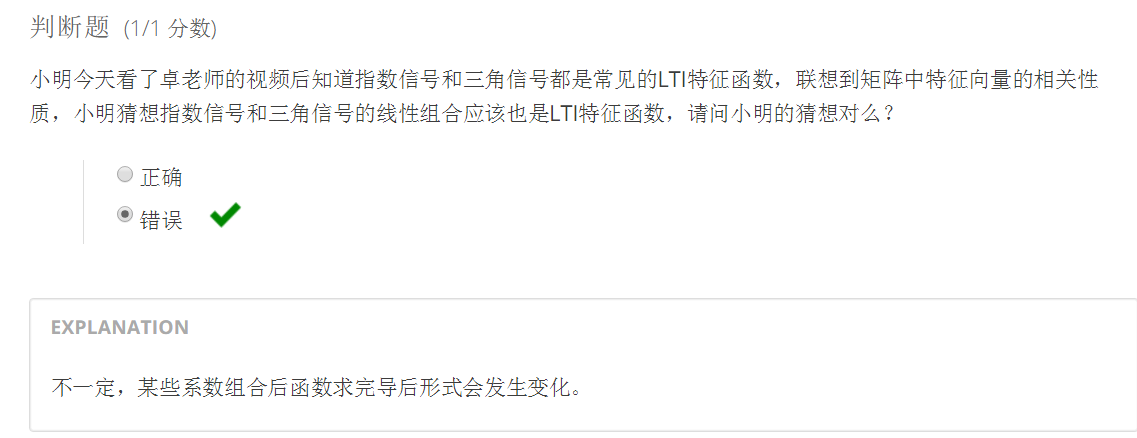展开全文• 典型雷达信号的波形仿真和分析.pdf典型雷达信号的波形仿真和分析.pdf典型雷达信号的波形仿真和分析.pdf典型雷达信号的波形仿真和分析.pdf典型雷达信号的波形仿真和分析.pdf典型雷达信号的波形仿真和分析.pdf典型雷达...
• 典型雷达信号的波形仿真和分析.docx典型雷达信号的波形仿真和分析.docx典型雷达信号的波形仿真和分析.docx典型雷达信号的波形仿真和分析.docx典型雷达信号的波形仿真和分析.docx典型雷达信号的波形仿真和分析.docx...
• Chirp信号的简介 Chirp信号是一个典型的非平稳信号，在通信、声纳、雷达等领域具有广泛的应用。 这是一个matlab对chirp信号的仿真实验
• 典型题解——第12章 非正弦周期电流电路和信号的频谱
• 利用MATLAB分析典型输入信号对稳态误差的影响.pdf
• 首先分析了Synchro仿真系统中信号配时优化模型、用于延误计算的百分比延误方法（PDM）模型和服务水平模型，并在调查实际数据的基础上对典型十字型交叉口的当前信号配时方案进行优化研究，同时提出将改善几何条件和...
• 高清原版PDF。 信号与系统考研典型题详解，涵盖多年各学校考研真题解析
• 信号与系统考研典型题详解，涵盖多年各学校考研真题解析
• ## 第三章：3.6 典型信号傅里叶变换

万次阅读 多人点赞 2017-08-25 16:33:22
关于对称性，我们傅里叶变换的对称性可以容易的得出结论，不要忘了这里的f（w）是偶函数。广义傅里叶变换如图所示，这是我们举的一个傅里叶变换的例子，对于单位阶跃信号没有办法直接去求傅里叶变换我们可以先通
• 什么是指数信号？ 解释：我们在中学就知道指数函数，而我在这里所说的指数信号则是 当 a=0 时，信号不随时间变化，成为直流信号 当 a>0 时，信号将随时间增长。 当 a<0 时，信号将随时间衰减。 常数 K...连续时间信号
• 典型数字信号处理应用中关键算法的硬件加速技术研究.pdf
• matlab_典型宽带信号处理方法，频率聚焦技术csm，宽带信号频率范围100-3100hz，对远场两个宽带信号源进行定位 Typical wideband signal processing method, frequency focusing technology, CSM, wideband signal ...
• 2018H1-2019H1中国4G智能手机在典型信号条件下平均语音MOS.xls行业数据
• 单边指数信号 我们设单边指数信号的表达式为 其中 为正实数。则 得 双边指数信号 设双边指数信号的表达式为非周期信号 傅里叶变换
• 一种新发现类似NMR现象的瞬态吸收信号典型特征，李文，，本文报道一种类似NMR的新的瞬态吸收信号现象并分析给出其二条基本典型特征：一条是信号主频率强度随时间变化的U型曲线，另一条是�...